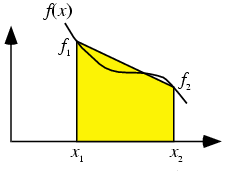•http://facebook.com/
•https://www.google.com/accounts/o8/id
•https://me.yahoo.com
COST (GBP)2.000.000

# trapezoidal

Computes the definite integral of a function using the trapezoidal rule.
Controller: CodeCogsC++
Excel

## Overview

This module computes the area beneath either a user supplied function or a set of discrete points, using an approximation which assumes the function is linear between each two consecutive points.

Consider a two times differentiable function , two distinct abscissas and a positive integer . Then the following approximation holds:

with an error bound of:

This is a quadrature formula known as the trapezoidal rule. From the formula of the error bound you may notice that e.g. if one doubles the number of points , the approximation error is decreased four times. To better understand how this rule works, observe the following picture - the area between abscissas and is approximated by a trapezoid.Below you will find examples that show how to use each version of the function and the error estimates in each case.

## References:

### Authors

Lucian Bentea (September 2006)

## Trapezoidal

 doubletrapezoidal( int n double (*f)(double)[function pointer] double a double b )
This version is to be used when you wish to pass the function to integrate as an argument, instead of passing an array with its values at distinct points.

### Example 1

In what follows an approximation is found for the definite integral and the absolute error from its actual value is estimated.
#include <codecogs/maths/calculus/quadrature/trapezoidal.h>
#include <stdio.h>
#include <math.h>

// number of points
#define N 100

// function to integrate
double f(double x)
{
return sin(x);
}

// the primitive of f, to estimate errors
double pf(double x)
{
return -cos(x);
}

int main()
{
// compute the approximate area
double fi = Maths::Calculus::Quadrature::trapezoidal(N, f, 1, 3),

// use the Leibniz-Newton formula to find a more precise estimate
realfi = pf(3) - pf(1);

// display problem data
printf("      f(x) = sin(x)\n");
printf("    points = %d\n\n", N);

// display the result and error estimate
printf("   I(1, 3) = %.15lf\n", fi);
printf("real value = %.15lf\n", realfi);
printf("     error = %.15lf\n\n", fabs(fi - realfi));

return 0;
}

### Output

f(x) = sin(x)
points = 100

I(1, 3) = 1.530243792301766
real value = 1.530294802468585
error = 0.000051010166819

### Parameters

 n the number of sample points of the function f, from which to approximate f the function to integrate a the inferior limit of integration b the superior limit of integration

### Returns

The definite integral of the given function from a to b.
##### Source Code

Source code is available when you buy a Commercial licence.

Not a member, then Register with CodeCogs. Already a Member, then Login.

## Trapezoidal

 doubletrapezoidal( int n const double* values double a double b )
This version is to be used when the function is not known analytically, but a table with its values at equally spaced abscissas is available.

### Example 2

The code below gives an approximation for the definite integral and estimates the absolute error from its actual value.
#include <codecogs/maths/calculus/quadrature/trapezoidal.h>
#include <stdio.h>
#include <math.h>

// the primitive of f, to estimate errors
double pf(double x)
{
return 2*sqrt(x*x*x)/3;
}

int main()
{
// values of the function at equally spaced abscissas
double ordinates = {1, 1.0247, 1.04881, 1.07238,
1.09544, 1.11803, 1.14017},

// compute the approximate area
fi = Maths::Calculus::Quadrature::trapezoidal(7, ordinates, 1, 1.3),

// use the Leibniz-Newton formula to find a more precise estimate
realfi = pf(1.3) - pf(1);

// display problem data
printf("      f(x) = sqrt(x)\n");
printf("    points = 7\n\n");

// display the result and error estimate
printf(" I(1, 1.3) = %.15lf\n", fi);
printf("real value = %.15lf\n", realfi);
printf("     error = %.15lf\n\n", fabs(fi - realfi));

return 0;
}

### Output

f(x) = sqrt(x)
points = 7

I(1, 1.3) = 0.321472250000000
real value = 0.321485368419253
error = 0.000013118419253

### Parameters

 n the number of sample points of the function f, from which to approximate values an array with the values of the function at equally spaced abscissas a the inferior limit of integration b the superior limit of integration

### Returns

The definite integral of the given function from a to b.
##### Source Code

Source code is available when you buy a Commercial licence.

Not a member, then Register with CodeCogs. Already a Member, then Login.